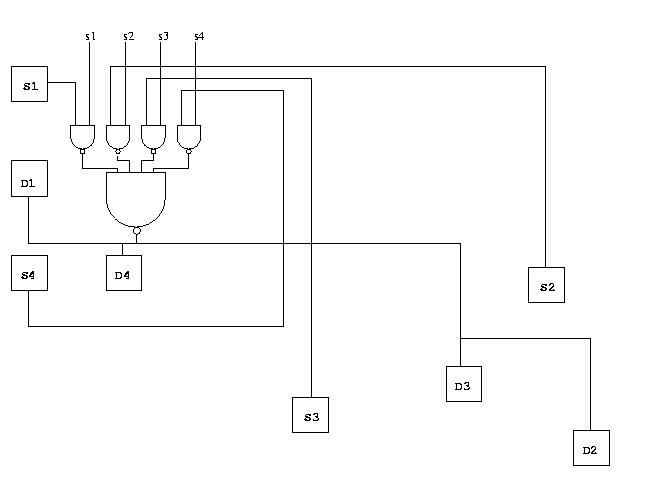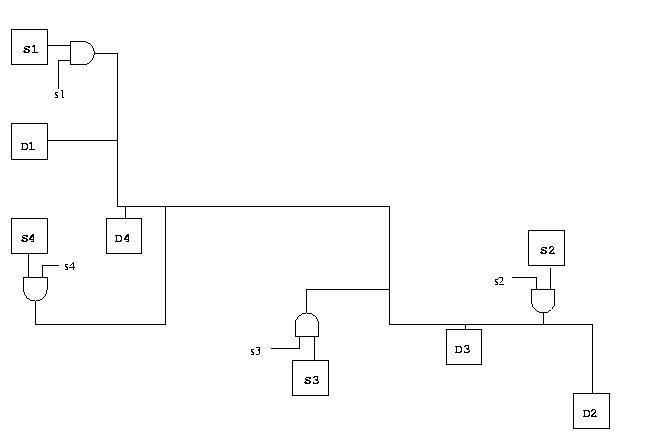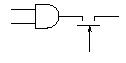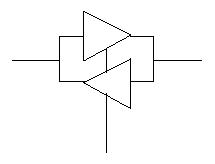Home  Products Tutorials Schematics Robotics Resources  Radio Stuff  Components Career  Download   Link Exchange   Sitemap

 Add your Link (FREE) Schematics and Circuits Electronic Tutorials Practical Experiments More Resources

Computer Architectures - Digital Circuits - Tri-state logic circuits

 Both combinatorial circuits and sequential circuits are fairly easy to understand, since we pretty much only have to understand logic gates and how they are interconnected. With tri-state logic circuits, this is no longer true. As their names indicate, they manipulate signals that can be in one of three states, as opposed to only 0 or 1. While this may sound confusing at first, the idea is relatively simple. Consider a fairly common case in which there are a number of source circuits S1, S2, etc in different parts of a chip (i.e., they are not real close together). At different times, exactly one of these circuit will generate some binary value that is to be distributed to some set of destination circuits D1, D2, etc also in different parts of the chip. At any point in time, exactly one source circuit can generate a value, and the value is always to be distributed to all the destination circuits. Obviously, we have to have some signals that select which source circuit is to generate information. Assume for the moment that we have singals s1, s2, etc for exactly that purpose. One solution to this problem is indicated in this figure:As you can see, this solution requires that all outputs are routed to a central place. Often such solutions are impractical or costly. Since only one of the sources is "active" at one point, we ought to be able to use a solution like this:However, connecting two or more outputs together is likely to destroy the circuits. The solution to our problem is to use tri-state logic. Return to the transistors A tri-state circuit (combinatorial or sequential) is like an ordinary circuit, except that it has an additional input that we shall call enable. When the enable input is 1, the circuit behaves exactly like the corresponding normal (not tri-state) circuit. When the enable input is 0, the outputs are completely disconnected from the rest of the circuit. It is as if there we had taken an ordinary circuit and added a switch on every output, such that the switch is open when enable is 0 and closed if enable is 1 like this:which is pretty close to the truth. The switch is just another transistor that can be added at a very small cost. Any circuit can exist in a tri-state version. However, as a special case, we can convert any ordinary circuit to a tri-state circuit, by using a special tri-state combinatorial circuit that simply copies its inputs to the outputs, but that also has an enable input. We call such a circuit a bus driver for reasons that will become evident when we discuss buses. A bus driver with one input is drawn like this: Here is a version for bidirectional signals:Terms & Conditions  Privacy Policy and Disclaimer
webmaster@electronicsteacher.com